CREW offers Open Access

CREW is in continuous Open Access phase to support your experiments free of charge!

Final public event & Globecom tutorial

CREW will present its final results at the Wireless Community event (Leuven, Belgium, 29 October 2015, more info) and organises a hands-on tutorial at Globecom (San Diego, USA, 10 December 2015, more info)

CREW PORTAL: access the CREW facilities

Interested in using the CREW facilities?
[Start here] - [Browse by name] - [Overview images] - [Advanced info] - [WTA GitHub].

Benchmarking and result analysis

Most of the time the steps involved in wireless experimentation are predefined. Start by defining an experiment, execute the experiment, analyze and compare the result. This tutorial explains the last part which is result analysis and performance (aka score) comparison. In the result analysis part, different performance metrics are graphically analyzed. For example, application throughput or received datagram from two experiments can be graphically viewed and compared. In performance comparison part, we do mathematical analysis on a number of performance metrics and come up with objective scores (i.e. 1 to 10). Subjective scores can also be mapped to different regions of the objective score range (i.e. [7-10] good, [4-6] moderate, [1-3] bad) but it is beyond the scope of this tutorial.

Coming back to our tutorial, we were at the end of experiment execution tool where we saved the experiment traces into a tar ball package. Inside the tar ball package there is database.tar file containing all SQLite database files, exprSpec.txt file which holds the experiment specific details and an XML metaData.xmlfile containing the output format of the database files stored. For this tutorial we use three identical optimization problem packages (i.e. Solution_ONE,Solution_TWOSolution_THREE). Download each package (i.e. located at the end this page) into your personal computer.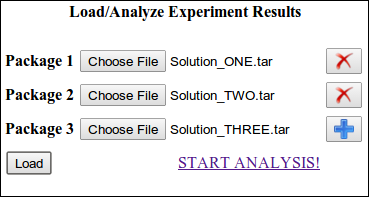Figure 1. result analysis and performance comparison tool at glance.

Result Analysis Section

Using the result analysis section, we visualize and compare the bandwidth of Node3 as seen by Node2 for different experiment runs. Click the ADD Graph button as shown in figure 1 above. It is also possible to create as many graphs as needed. Each graph is customized by three subsections. The first is database selection. Here we select different experiment runs that we need to visualize. For this tutorial, three distinct solutions were conducted in search of the optimal bandwidth over the link Node3 to Node2. Figure 2 shows the database selection view of the ISR experiment.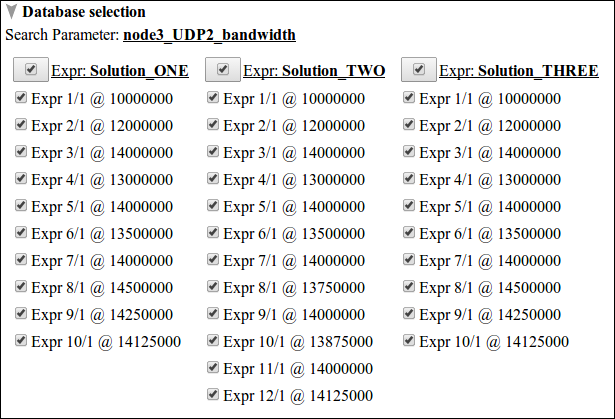Figure 2. database selection view of the ISR experiment over three distinct solutions.

From the figure above, the search parameter node3_UDP2_bandwidth indicates the tune variable that was selected in the parameter optimization section of experiment execution tool. Moreover, The number of search parameters indicates the level of optimization carried, thus for ISR experiment it is a single parameter optimization problem. The other thing we see on the figure is solutions are grouped column wise and each experiment is listed according to experiment #, run # and specific search parameter value. For example Expr 10/1 @ 13875000 indicates experiment # 10, run # 1 and node3_UDP2_bandwidth=13875000 bit/sec. Before continuing to the other subsections, deselect all experiment databases and select only Expr 1/1 @ 10000000 from all solutions.

The second subsection, graph analysis, selects x-axis and y-axis data sets. To this end, the database meta data file is parsed into structured arrangement of groups, applications, measurement points, and metrics out of which the data sets are queried. Besides this, there are three optional selection check-boxes; AVG/STDEVLABEL, and RANGE.  AVG/STDEV is used to enable average and standard deviation plotting over selected experiments. LABEL and RANGE turns on and off plot labeling and x-axis y-axis range selection respectively.

The last subsection is custom graph analysis and it has similar function like the graph analysis subsection. Compared to the graph analysis subsection, SQL statements are used instead of parsed XML metadata to define x-axis and y-axis data sets. This gives experimenters the freedom to customize a wide of range of visualizations. Figure 3 shows graph analysis and custom graph analysis subsections of the ISR experiment. For graph analysis, begin_interval and size (i.e. bandwidth) from Node2 group, iperf_UDP application, transfer measurement point are used as x-axis and y-axis data sets respectively. For custom graph analysis, mean interval and size (i.e. bandwidth) are used as x-axis and y-axis data sets respectively.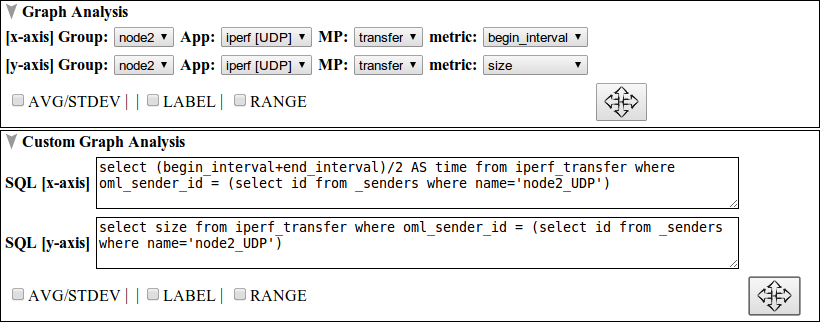Figure 3. graph analysis and custom graph analysis subsection view.

Now click the arrow crossed button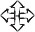in either of the graph analysis subsections to visualize datagram plot for the selected experiments. Figure 4 shows such a plot for six experiments.Figure 4. bandwidth vs. time plot for three identical experiments.

The first thing we see from the above figure is in each of the three experiments, Node2 reported almost identical bandwidth over the one minute time interval.Second the y-axis plot is zoomed within maximum and minimum result limits. Sometimes, however, it is interesting to see bandwidth plot over the complete y-axis range which is starting from zero up to the maximum. Click the RANGE check box and fill in 0 to 55 in the x-axis range and 0 to 1500000 in the y-axis range. Moreover, in repeated experiments one of the basic things is visualizing the average pattern of repeated experiments and see how much deviation each point has from average. Check the AVG/STDEV check-box (check the SHOW ONLY AVG/STDEV check-box if only AVG/STDEV plot is needed). Figure 5 shows the final modified plot when complete y-axis range and only AVG/STDEV plot is selected.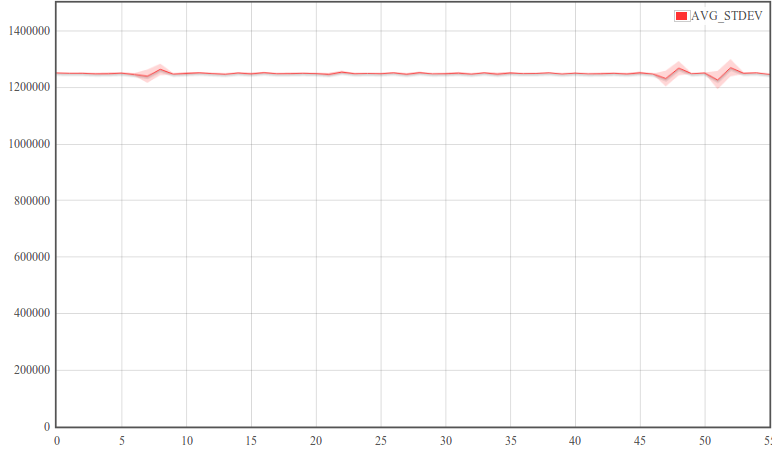Figure 5. average bandwidth as a function of time plot from three identical experiments

Performance comparison Section

In performance comparison, a number of experiment metrics are combined and objective scores are calculated in order to compare how good or bad an experiment performs related to other experiments.

For this part of the tutorial, we create a simple score out of 100 indicating how much percent the receive bandwidth reaches and the transmit bandwidth. The higher the score the better the receive bandwidth approaches the transmit bandwidth and vise versa. Start by clicking the ADD Score button and a score calculation block is created ( Note: go further down to reach the score calculation section). A score calculation block has three subsections namely variable definition, score evaluation and database selection.

The variable definition subsection defines SQL driven variables that are used in the score evaluation process. For this tutorial, we create two variables one evaluating the average receive bandwidth and the second evaluating the transmit bandwidth. Click the plus icon twice and enter the following SQL command into the first text-area "SELECT avg(size) from iperf_transfer where oml_sender_id=(select id from _senders where name='node2_UDP')". For the second variable click the icon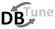to select variables from search parameters and select node3_UDP2_bandwidth.

The next subsection, score evaluation, is a simple mathematical evaluator with built in error handling functionality. Coming back to our tutorial, to simulate the percentage of receive bandwidth to transmit bandwidth, enter the string 100*(8*x1)/x2 in the score evaluation text-area ( Notex1 is multiplied by 8 to change the unit from byte/sec to bit/sec).

Finally, database selection subsection serves the same purpose as was discussed in the result analysis section. Now press the arrow crossed button in the score evaluation subsection. Figure 6 shows the scores evaluated for the ISR experiment.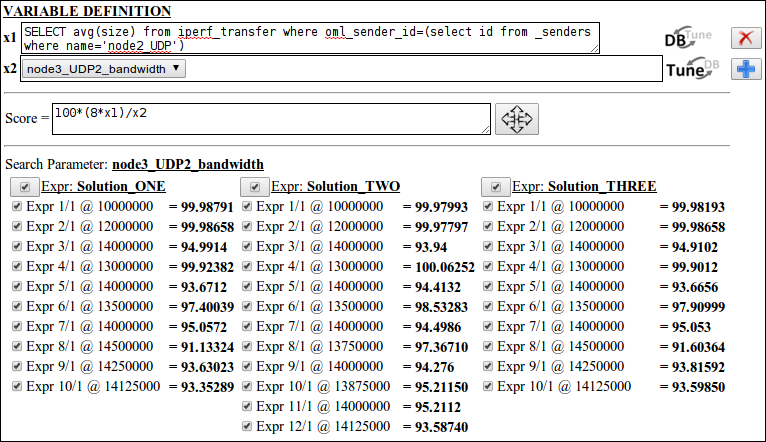Figure 6. score evaluation section showing different configuration settings and score results for the ISR experiment.

Performance comparison is possible for identical search parameter settings among different solutions. For example comparing Expr1/1 @ 10000000 experiments from the three solutions reveals that all of them have receive bandwidth about 99.98% of the transmit bandwidth and thus they are repeatable experiments. On the other hand, the scores from any Solution show the progress of the optimization process. Recall the objective function definition (i.e. (8*x1)/x2 < 0.95 => stop when 8*x1 < 0.95*x2) in the ISR experiment such that the IVuC optimizer triggers the next experimentation cycle only when the receive bandwidth is less than 95% of the transmit bandwidth. Indeed on the figure above, search bandwidth decreases as the performance score decreases below 95%. Therefore performance scores can be used to show optimization progress and compare among different Solutions.

AttachmentSize
Solution_ONE.tar380 KB
Solution_TWO.tar450 KB
Solution_THREE.tar380 KB